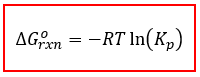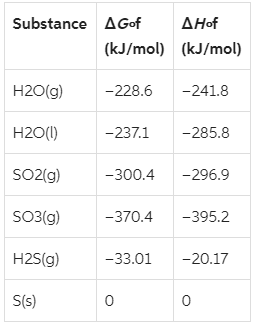# Problem: The reactionSO2(g) + 2H2S(g) ⇌ 3 S(s) + 2H 2O(g)is the basis of a suggested method for removal of SO 2, a pollutant that irritates airways causing coughing, from power-plant stack gases. The values below may be helpful when answering questions about the process.Calculate the equilibrium constant Kp for the reaction at a temperature of 298 K. Express the equilibrium constant to one significant figure.

###### FREE Expert Solution

Based on the reaction for the removal of SO2, and the given data values of Δf and Δf for reactants and products, we’re asked to determine Kp of the reaction at a temperature of 298 K.

Remember that Kp is the Equilibrium constant in terms of pressure.

Additionally, Kp is related to the standard Gibbs free energy (ΔG°rxnby the following equation:Where

Δrxn is the Gibbs energy of the reaction

R is the gas constant (R = 8.314 J/molK)

T is the temperature (in K)

Kp is the equilibrium constant in terms of pressure.

93% (439 ratings)###### Problem Details

The reaction

SO2(g) + 2H2S(g) ⇌ 3 S(s) + 2H 2O(g)

is the basis of a suggested method for removal of SO 2, a pollutant that irritates airways causing coughing, from power-plant stack gases. The values below may be helpful when answering questions about the process.Calculate the equilibrium constant Kp for the reaction at a temperature of 298 K. Express the equilibrium constant to one significant figure.